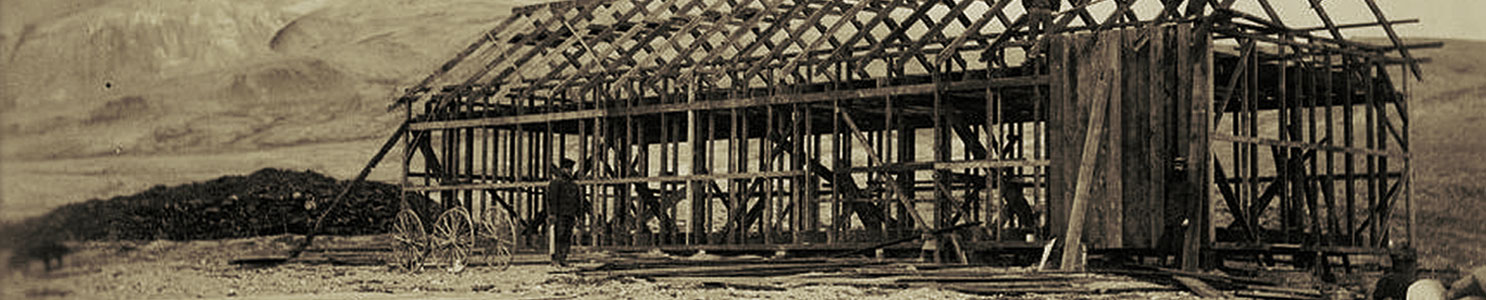# Measuring Gravity at Fort Conger

It was known by scientists that every object in the universe attracts every other object. This phenomenon is called gravitation and the force of attraction is commonly known as gravity. In the case of our planet earth, (terrestrial) gravity is the force of attraction between the earth and an object on, below or above the surface of the earth. Because the earth is an oblate spheroid, being slightly flattened at the poles due to the earth’s rotation, the gravitational force at the equator is slightly less than the gravitational force at the poles.
But is it possible to measure the differences that such changes in latitude produce in the force of gravity?

## How it Works: Take a Kater’s Pendulum for a Swing!

The Peirce No.1 Pendulum used at Fort Conger had a heavy and a light end. In order to calculate the force of gravity, the pendulum’s period of oscillation (number of swings over a specified period of time) had to be identical when suspended with either the heavy or light end facing down.

Instructions: Click on the Options button and select Play! In this game you have four different cases. There is a start button for each case. Click the button to start the stopwatch and you will see, through the telescope lens, the oscillations (swings) of the pendulum. Swing the pendulum with its heavy end down. After 10 oscillations, the timer will stop and the measurements will be revealed to you. Next, flip the pendulum so that its light end is pointing down and repeat the experiment. Did the heavy and light end times match over 10 swings? If not, select another case and try again.

Note: The measurements T and L are crucial for determining the force of gravity (gravitational constant [G]) acting on the pendulum. L is the length of the pendulum below the point of suspension. T = 2pi sq. root of (L/G).